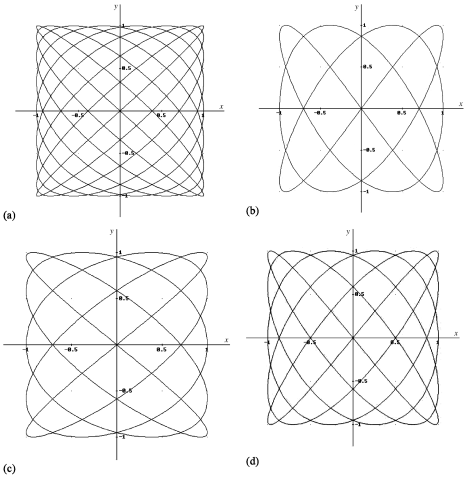/
/
/
4.A nice family of Lissajous curves generated by taking a
Not my Question
Flag Content

# Question : 4.A nice family of Lissajous curves generated by taking a : 1710548

4.A nice family of Lissajous curves is generated by taking a to be an odd integer andb = a ± 1. Match the following parametric equations with the curves in Figure 2.

A: x = cos 5t, y = sin 4t   B: x = cos 3t, y = sin 4t

C: x = cos 5t, y = sin 6t   D: x = cos 9t, y = sin 8tFigure 2

5. Without graphing, describe the curve produced by the equations x = cos 10t, y = sin 10t.

6. What is the effect of introducing a phase angle in the equations? For example, plot and describe the curve=x = cos (5t + π/4), y = sin 4t, in which the x-coordinate has the phase angle π/4.

7. Graph the curve described by the equationsx = cos 2t, y = sin t. Describe this curve in terms of x and y.

## Solution 5 (1 Ratings )

Solved
Mathematics 2 Days Ago 4 Views